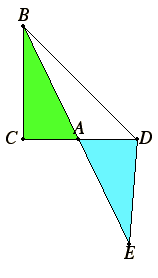# Proposition 5

In equal triangles which have one angle equal to one angle the sides about the equal angles are reciprocally proportional; and those triangles which have one angle equal to one angle, and in which the sides about the equal angles are reciprocally proportional, are equal.

Let ABC and ADE be equal triangles having one angle equal to one angle, namely the angle BAC equal to the angle DAE.

I say that in the triangles ABC and ADE the sides about the equal angles are reciprocally proportional, that is to say, that CA is to AD as EA is to AB.I.14

Place them so that CA is in a straight line with AD. Therefore EA is also in a straight line with AB.

Join BD.

V.7

Since then the triangle ABC equals the triangle ADE, and ABD is another triangle, therefore the triangle ABC is to the triangle ABD as the triangle ADE is to the triangle ABD.

VI.1

But ABC is to ABD as AC is to AD, and ADE is to ABD as AE is to AB.

V.11

Therefore also AC is to AD as AE is to AB.

Therefore in the triangles ABC and ADE the sides about the equal angles are reciprocally proportional.

Next, let the sides of the triangles ABC and ADE be reciprocally proportional, that is to say, let AE be to AB as CA is to AD.

I say that the triangle ABC equals the triangle ADE.

If BD is again joined, since AC is to AD as AE is to AB, while AC is to AD as the triangle ABC is to the triangle ABD, and AE is to AB as the triangle ADE is to the triangle ABD, therefore the triangle ABC is to the triangle ABD as the triangle ADE is to the triangle ABD.

Therefore each of the triangles ABC and ADE has the same ratio to ABD.

V.9

Therefore the triangle ABC equals the triangle ADE.

Therefore, in equal triangles which have one angle equal to one angle the sides about the equal angles are reciprocally proportional; and those triangles which have one angle equal to one angle, and in which the sides about the equal angles are reciprocally proportional, are equal.

Q.E.D.

## Guide

This proposition is used in the proof of proposition VI.19.